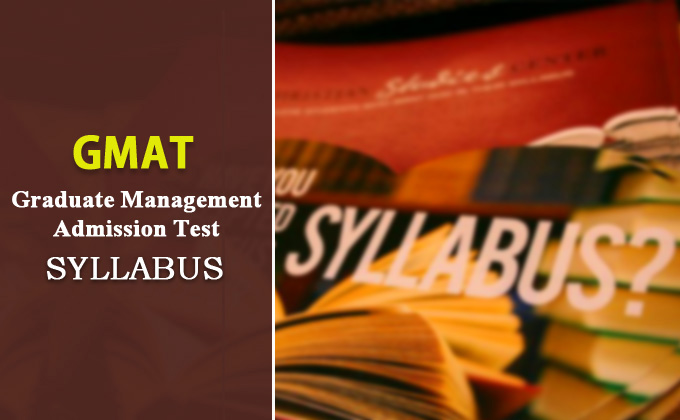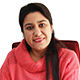## I. Quantitative Assessment Section: Problem solving & Data Sufficiency

There will be 19 questions of problem solving type and 18 questions from data sufficiency type.

This section aims to measure the ability to reason quantitatively, solve quantitative problems and interpret data.

## Tips for Data Sufficiency problems:

1. Read the statements separately and write the fine points. Never read the statements together.

3. If you don’t have fair idea of the answers then elimination method may work in your favor provided you eliminate minimum two options.

 Syllabus : QA I Arithmetic 1 Number System 2 HCF and LCM 3 Powers Roots of Numbers 4 Ratio and Proportion 5 Partnership and Share 6 Averages 7 Percentages 8 Profit and Loss 9 Simple and Compound Interest 10 Time and Work 11 Time and Distance II Algebra 1 Algebraic Expressions and Operations 2 Polynomials 3 Factorizations 4 Quadratic Polynomials 5 Cubic Polynomials 6 System of Equations 7 Sequence and Series III Geometry 1 Lines and Angles 2 Triangles 3 Polygons 4 Quadrilaterals 5 Circles 6 Cube and Cuboids 7 Cylinders and Cones 8 Spheres and Hemispheres IV Statistics & Probability 1 Classification of Data, Frequency and Range 2 Mean, Median and Mode 3 Variance and Standard Deviations 4 Discrete Probability 5 Addition and Multiplication Theorems in Probability V Modern Math 1 Sets, Relation & Functions 2 Permutation and Combination

## II. Integrated Reasoning Section:

This section was added in June 2012 to the GMAT. It is a combination of both verbal and quant. There will be 12 questions in IR section which consists of four types:

• Multi source reasoning
• Graphics interpretation
• Table analysis
• Two-part analysis

The main objective of the IR section:

• Ability to develop relevant information presented in text, numbers and graphics
• Ability to assess appropriate information from different sources
• Ability to combine and arrange information to observe relationships among them and solving complex problems to arrive at correct interpretation.

## III. Verbal Section:

There will be 41 questions in verbal section which consists of three types: